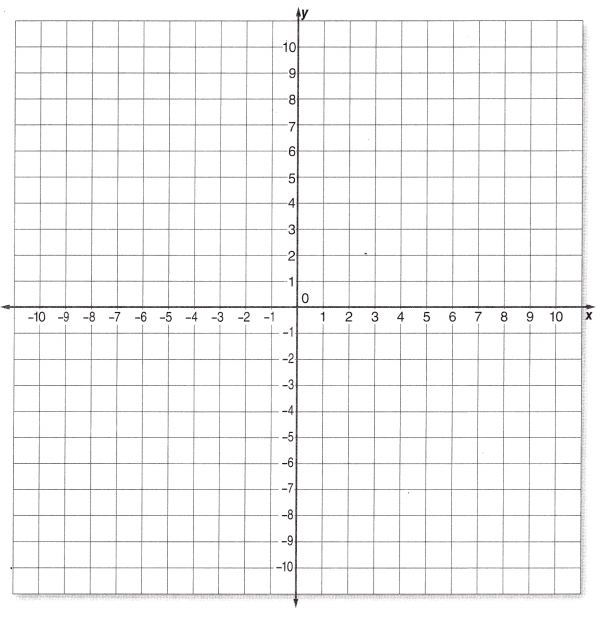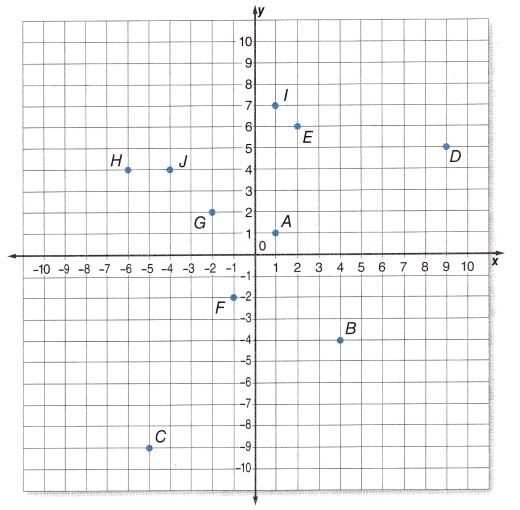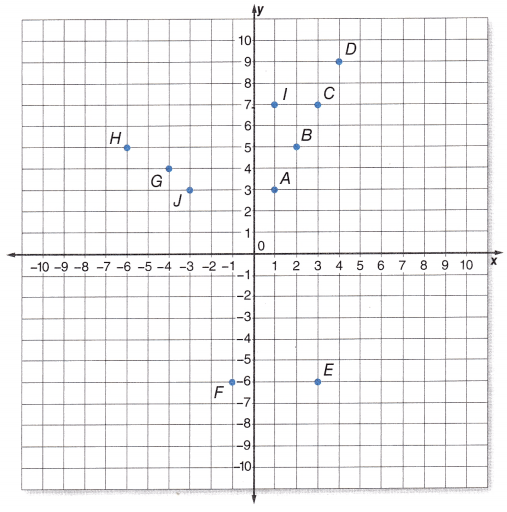# McGraw Hill Math Grade 8 Lesson 14.1 Answer Key Plotting Ordered Pairs

Practice the questions of McGraw Hill Math Grade 8 Answer Key PDF Lesson 14.1 Plotting Ordered Pairs to secure good marks & knowledge in the exams.

## McGraw-Hill Math Grade 8 Answer Key Lesson 14.1 Plotting Ordered Pairs

Exercises

GRAPH

Question 1.
Plot the following ordered pairs on the graph:A (4, 2)
B (6, -6)
C (-8, -8)
D (-4, 4)
E (5, 5)
F (2, 9)
G (3, 4)
H (-4, -3)
I (-5,-5)
J (6, -1)
K (-3, -3)Explanation:
Plotted the following ordered pairs A (4, 2), B (6, -6), C (-8, -8),
D (-4, 4), E (5, 5), F (2, 9), G (3, 4), H (-4, -3), I (-5,-5), J (6, -1), K (-3, -3)
on the graph as shown above.

Question 2.
Give the coordinates for each point on the graph:A _____________
B _____________
C _____________
D _____________
E _____________
F _____________
G _____________
H _____________
I _____________
J _____________
A (1, 1), B (4, -4), C (-5, -9), D (9, 5), E (2, 6), F (-1, -2), G (-2, 2),
H (-6, 4), I (1,7), J (-4, 4),

Explanation:
The coordinates for each point on the graph are A (1, 1), B (4, -4), C (-5, -9), D (9, 5), E (2, 6), F (-1, -2), G (-2, 2), H (-6, 4), I (1,7), J (-4, 4).

Question 3.
Give the coordinates for each point on the graph:A _____________
B _____________
C _____________
D _____________
E _____________
F _____________
G _____________
H _____________
I _____________
J _____________